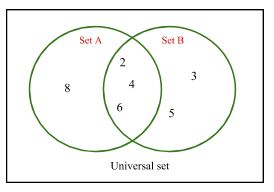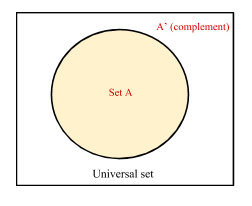# Set Theory

Contents

## Summary

• The modern study of set theory was initiated by Georg Cantor and Richard Dedekind in the 1870s.
• A set is a collection of distinct objects, called elements of the set.
• Set’s are denoted by upper case letters and they are enclosed in curly brackets  {….}.
• When we write$x\quad \in \quad A$, this means x belongs to the set A.
• An empty set is denoted by ∅.
• Subset contains fewer or all elements of only its parent set and it is denoted by the symbol ⊆. If B is a subset of A, we write BA.
• Some important set operations are:

Union: A ⋃ B
Intersection: A ⋂ B
Complement: Complement of set A is written as${ A }^{ c }$

The modern study of set theory was initiated by Georg Cantor and Richard Dedekind in the 1870s. Set theory includes a diverse collection of topics, ranging from the structure of the real number line to the study of the consistency of large cardinals.

Set Theory is fundamental to modern mathematics. It is the alphabet of mathematics.

#### What is a set?

A set is a collection of distinct objects, called elements of the set. When we know all members of the set, it is known as definite set.

Set’s are denoted by upper case letters (variables) and the elements of the set are denoted by lower case letters. These are enclosed in curly brackets.

We can say two sets are equal if they have the same elements. The symbol$\in$  means ”is an element of”. I.e when we write$x\quad \in \quad A$,  this means x belongs to the set A.

The set that contains no elements is the empty set, and is denoted by ∅. Some examples of sets are listed below:

• {1, 2, 3, 4}
• {cat, dog, bird, fish}
• {orange, yellow, blue, green, white}
• {50, 55, 86, 88, 89, 90}

Some of the sets that have special notations for different types are:

N = Set of Natural Numbers
Q = Set of Rational Numbers
R = Set of Real Numbers
P = Set of Prime Numbers
Z = Set of Integers
E = Set of Even Integers
O = Set of Odd Integers

#### What is a Subset?

Subset contains fewer or all elements of only its parent set. For example if it is said that B is a subset of A, then this means all of the elements of B are also in A. Symbol ⊆ is used to denote a subset.

If B is a subset of A, we write B ⊆ A. i.e

A = {5, 10, 15, 20, 25}
B = {10 ,15}

We can see that B is a subset of A written as B ⊆ A.

#### Venn Diagrams

A very common method to represent operations of sets are through venn diagrams. This way we can easily visualise the relation between different sets.
For example we can represent set A={5, 10, 15, 20, 25} through a venn diagram in the following way.Note that set A (the circle) is a subset of the Universal set (the rectangle).

Some important set operations are:

i) Union

The union of two sets contains all the elements contained in either set (or both sets).

The union is notated A ⋃ B.

For example if we have two sets A = {2, 4, 6, 8} and B = (2, 3, 4, 5, 6}, then

A ⋃ B ={2, 3, 4, 5, 6, 8} note we list 2, 4, 6 only once.

This can be shown on a venn diagram in the following way:ii) Intersection

The intersection of two sets contains only the elements that are in both sets.

The intersection is notated A ⋂ B.

For example if we have two sets A = {2 , 4, 6, 8} and B = (2, 3, 4, 5, 6}, then

A ⋂ B = {2, 4, 6}.

This can be shown on a venn diagram in the following way:iii) Complement

The complement of a set A contains everything that is not in the set A.

The complement is notated A’, or${ A }^{ c }$.

A complement is relative to the universal set, so${ A }^{ c }$  contains all the elements in the universal set that are not in A.